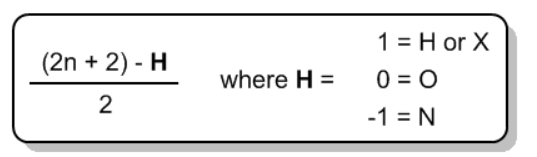# Problem: Calculate the unsaturation number for each of the following compounds:a. C5H8N2b. C5H9N

###### FREE Expert Solution

We are going to use the formula below to calculate degrees of unsaturation:Where: n = number of carbon atoms

a. C5H8N2

$\frac{\left(2n+2\right)\mathbf{-}\mathbf{H}}{\mathbf{2}}\phantom{\rule{0ex}{0ex}}=\frac{\mathbf{\left(}\mathbf{2}\left(5\right)\mathbf{+}\mathbf{2}\mathbf{\right)}\mathbf{-}\left(8-2\right)}{\mathbf{2}}\phantom{\rule{0ex}{0ex}}=\frac{\mathbf{\left(}\mathbf{10}\mathbf{+}\mathbf{2}\mathbf{\right)}\mathbf{-}\mathbf{\left(}\mathbf{6}\mathbf{\right)}}{\mathbf{2}}\phantom{\rule{0ex}{0ex}}=\frac{\mathbf{\left(}\mathbf{12}\mathbf{\right)}\mathbf{-}\mathbf{\left(}\mathbf{6}\mathbf{\right)}}{\mathbf{2}}\phantom{\rule{0ex}{0ex}}=\frac{\mathbf{6}}{\mathbf{2}}$

100% (5 ratings)###### Problem Details

Calculate the unsaturation number for each of the following compounds:

a. C5H8N2

b. C5H9N